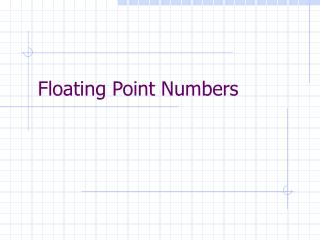# Floating Point Numbers - PowerPoint PPT PresentationDownload PresentationFloating Point Numbers

Floating Point NumbersDownload Presentation## Floating Point Numbers

- - - - - - - - - - - - - - - - - - - - - - - - - - - E N D - - - - - - - - - - - - - - - - - - - - - - - - - - -
##### Presentation Transcript

1. Floating Point Numbers

2. It's all just 1s and 0s • Computers are fundamentally driven by logic and thus bits of data • Manipulation of bits can be done incredibly quickly • Given n bits of information, there are 2n possible combinations • These 2n representations can encode pretty much anything you want, letters, numbers, instructions….

3. Bases of number systems • Base 10 numbers: 0,1,2,3,4,5,6,7,8,9 • 3107 = 3103 +1102 + 0101 +7100 • Base 2 numbers: 0,1 • 3107 = 1 2 4 8 16 32 64 128 256 512 1024 2048 • =1211 + 1210 + 029 + 028 + 027 + 026 + 125 + 024 + 023 + 022 + 121 + 120 • =110000100011 • Addition, multiplication etc, all proceed same way

4. Base Notation • What does 10 mean? • 10 in binary = 2 decimal • 10 in octal (base 8) = 8 decimal • 10 in decimal = 10 decimal • Need some method of differentiating between these possibilities • To avoid confusion, where necessary we write • 1010= • 102=

5. Integer Representation • Integers obviously fit into this base 2 notations • Remains challenge to represent negative numbers • 2s complement • Excess-N • Extra choice is order of bits • Choice is made chip-by-chip • portability

6. Floating Point Representation • Computers represent oating point numbers in binary form • For generality, they use a binary form of scientic notation In binary, we can use powers of 2

7. Floating Point Size • In IEEE.h • IEEE.h:#define IEEE_FLOAT_SIZE 4 • IEEE.h:#define IEEE_DOUBLE_SIZE 8 • IEEE.h:#define IEEE_QUAD_SIZE 16

8. Distribution

9. In Decimal Terms • Each binary floating point double holds roughly 16 decimal digits • technically, 2^(-52) • MATLAB example

10. Advantages • Scientific notation can work on any scale (all handled by exponent) • So long as errors are small relative to scale of data values, calculations are accurate • right?

11. Example 1 • 1e12 + 0.2 – 1e12

12. Problem • Nice decimal numbers (0.2) have continuing binary representations • like 1/3 = 0.3333333, 0.2 has binary 0.0011 0011 0011 0011… • Analogy with adding, subtracting large number

13. Roundoff Error • Round-off error will always be present e.g. • Roundoff error is more significant when you are subtracting two almost equal quantities • e.g in decimal, 255.67 – 255.69

14. Example 2 • A = 112000000 • B = 100000 • C = 0.0009 • X = A - B / C

15. Common occurrence • Delta x in • finite element methods • numerical differentiation • Places where more closely packed data gives

16. Example 3: Numerical Diff.

17. Example 4: Recursion • Comparing sum of delta x and real sum • t = 0; • N = 10000; dx = 1/N; • for (I = 1:N) • t = t + dx; • end

18. Avoiding (Large) Roundoff Error • Avoid substracting almost-equal quantities • Avoid dividing by small quantities • Avoid sums over large loops, especially with different orders of magnitude in the sum • Avoid recursive calculations, where errors will accumulate